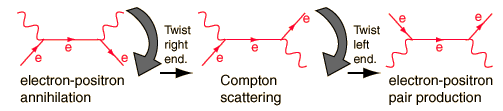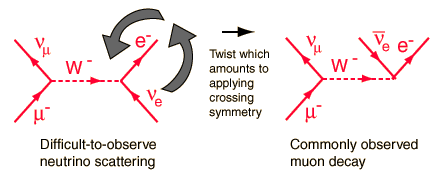# Twisted Feynman Diagrams and Crossing Symmetry

Once you have constructed a Feynman diagram for a particular particle interaction, you can then predict other interactions by rotating and/or twisting the diagram.This feature of Feynman diagrams is particularly useful for charged weak interactions. In that case interactions can be constructed from the weak interaction Feynman diagrams, but the interactions that are often observed are twisted versions of the interactions constructed from the primitive vertices. A good example is the development of the Feynman diagram for muon decay.From the primitive vertices we construct the interaction m, ne -> e, nm and then just twist the right end so that the electron neutrino component is an exit particle instead of an entering particle. The process turns the arrow downward, representing the antiparticle, the electron anti-neutrino. This is just the symmetry operation addressed in crossing symmetry. It is generally observed that if a given interaction exists, then the interaction constructed by taking a particle to the other side of the reaction and turning it into its antiparticle will also exist.

Index

Particle concepts

Reference
Griffiths
Ch 2

 HyperPhysics***** Quantum Physics R Nave
Go Back